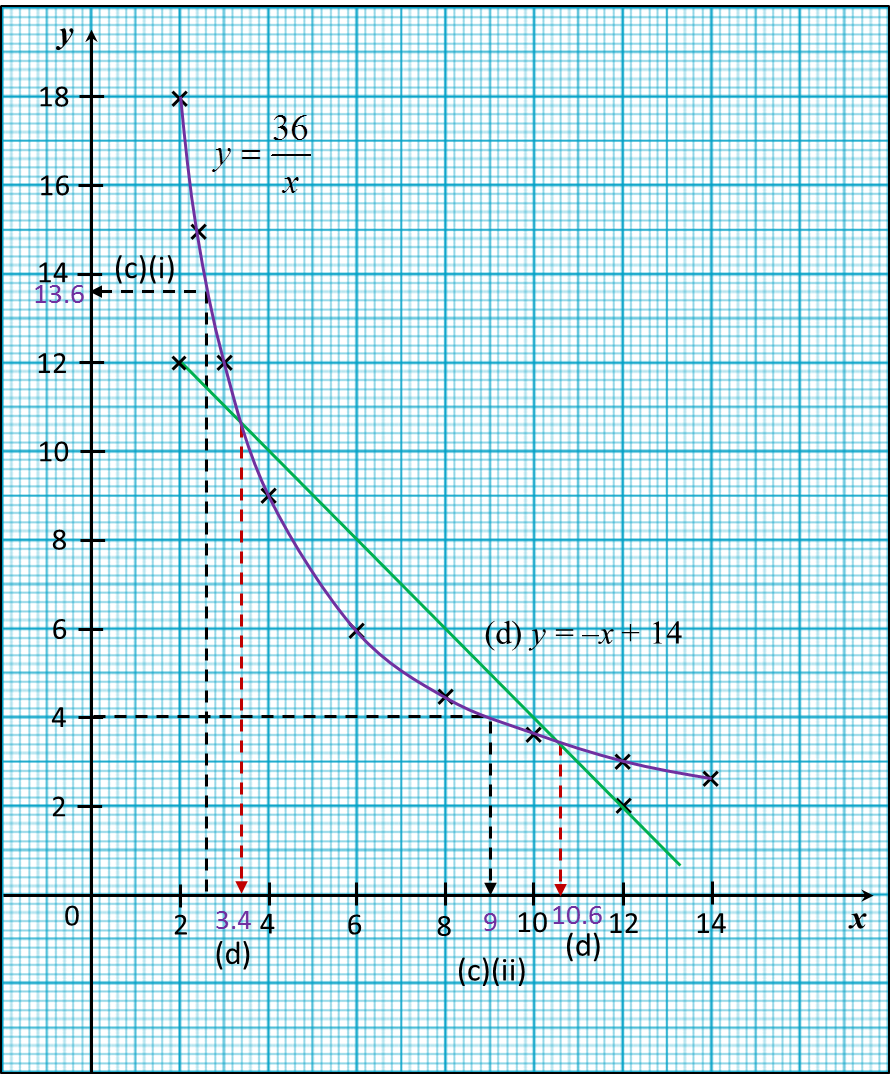# 2.5.2 Graph of Functions (II), SPM Paper 2 (Long Questions)

Question 5:
(a) The following table shows the corresponding values of x and y for the equation $y=\frac{24}{x}$

 x –4 –3 –2 –1 1 1.5 2 3 4 y –6 k –12 –24 24 n 12 8 6
Calculate the value of k and n.

(b) For this part of the question, use graph paper. You may use a flexible curve rule.
By using a scale of 2 cm to 1 unit on the x-axis and 2 cm to 5 units on the y-axis, draw the graph of  $y=\frac{24}{x}$  for –4 ≤ x ≤ 4.

(i) The value of when x = 3.5,
(ii) The value of when y = –17.

(d) Draw a suitable straight line on your graph to find the value of x which satisfy the equation 2x2 + 5= 24 for –4 ≤ x ≤ 4.

Solution:
(a)

(b)(c)
(i) From the graph, when x = 3.5, y = 7
(ii) From the graph, when y = –17, x = –1.4

(d)

$\begin{array}{l}\text{}y=\frac{24}{x}\text{-------------- (1)}\\ \text{}2{x}^{2}+5x=24\text{------ (2)}\\ \left(2\right)÷x,\text{}2x+5=\frac{24}{x}\text{-------- (3)}\\ \left(1\right)-\left(3\right),\text{}y-\left(2x+5\right)=0\\ \text{}y=2x+5\end{array}$

The suitable straight line is y = 2x + 5.
Determine the x-coordinate of the point of intersection of the curve and the straight line = 2x + 5.

 x 0 3 y = 2x + 5 5 11
From the graph, = 2.5

Question 6:
(a) Complete the table in the answer space for the equation $y=\frac{36}{x}$   by writing down the values of y when x = 3 and x = 8.

(b) For this part of the question, use graph paper. You may use a flexible curve rule.
By using a scale of 1 cm to 1 unit on the x-axis and 1 cm to 1 unit on the y-axis, draw the graph of $y=\frac{36}{x}$  for 2 ≤ x ≤ 14.

(i) the value of y when x = 2.6,
(ii) the value of x when y = 4.

(d) Draw a suitable straight line on your graph to find the values of x which satisfy the equation $\frac{36}{x}+x-14=0$  for 2 ≤ x ≤ 14.

 x 2 2.4 3 4 6 8 10 12 14 y 18 15 9 6 3.6 3 2.6

Solution:
(a)

(b)(c)
(i) From the graph, when x = 2.6, y = 13.6
(ii) From the graph, when y = 4, x = 9

(d)

The suitable straight line is y = x + 14.

 x 2 12 y = –x + 14 12 2
From the graph, x = 3.4, 10.6.